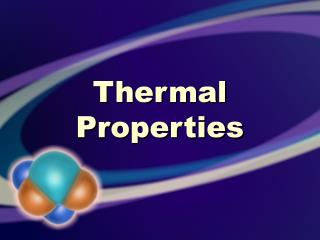# Thermal Properties - PowerPoint PPT PresentationDownload PresentationThermal Properties

Thermal PropertiesDownload Presentation## Thermal Properties

- - - - - - - - - - - - - - - - - - - - - - - - - - - E N D - - - - - - - - - - - - - - - - - - - - - - - - - - -
##### Presentation Transcript

1. Thermal Properties

2. Thermal Expansion • When a solid is heated, its atoms spread out in all dimensions. • Consequences of thermal expansion may be desirable or undesirable!

3. Thermal Expansion • To calculate a change in length due to thermal expansion: Δl = αliΔt

4. Thermal Expansion • α is the coefficient of thermal expansion • different for each material • units: °C-1 or K-1 Δl = αliΔt

5. Thermal Expansion • Δl and li must be in the same units of length • Δt and α must use the same temperature units Δl = αliΔt

6. Thermal Expansion • bimetallic strips take advantage of different rates of expansion of different materials • thermostats

7. Thermal Expansion • Expansion of volume can be similarly calculated: • β is the coefficient of volume expansion. • β = 3α ΔV = β ViΔt

8. Thermal Expansion • Liquids respond to temperature changes much more than solids do. • The same equation can be used for liquid expansion. ΔV = β ViΔt

9. Thermal Expansion • Example 14-3: • How much does the gasoline expand? • How much does the tank expand? • How much gas spills?

10. Thermal Expansion • Gases also expand in volume with changes in temperature; this is discussed in more detail later in the chapter.

11. Measuring Temperature

12. Thermometers • Galileo—thermoscope • drawback: also responded to changes in air pressure

13. Thermometers • Gas thermometers are more accurate but less convenient. • Now liquid thermometers use liquids sealed in glass tubes under vacuum.

14. Liquid Thermometers • must be calibrated • must be sensitive enough that a small change in temperature produces a noticeable change in liquid level

15. Liquid Thermometers • should respond quickly to changes in temperature • mass must be small in relation to the bulk of the object whose temperature is being measured

16. Liquid Thermometers • medium used in thermometer must be appropriate for the range of temperatures being measured

17. Thermometric • A thermometric property of a substance is any measurable property that changes in proportion to a corresponding change in temperature.

18. Thermometric • other temperature-measuring instruments: • resistive temperature detectors (RTD) • thermocouple junctions

19. Thermometric • other temperature-measuring instruments: • mechanical thermometers • digital thermometers

20. Temperature Scales • Fahrenheit • After trying other things, the reference points became 32° (freezing point of water) and 212° (boiling point of water).

21. Temperature Scales • Celsius • This scale sets the freezing point of water at 0° and the boiling point of water at 100°.

22. Temperature Scales • Absolute or Kelvin • emphasized an “absolute zero” • absolute zero is 0K or about -273.15°C

23. Temperature Scales • Absolute or Kelvin • also uses triple point of water as a reference • the degree symbol (°) is not used

24. Conversions • Celsius (tC) and Kelvin (T): • T = tC + 273.15 • tC = T – 273.15

25. Conversions • Fahrenheit (tF) to Celsius (tC): • tC = 5/9(tF + 40°) – 40° • tC = 5/9(tF – 32°)

26. Conversions • Celsius (tC) to Fahrenheit (tF): • tF = 9/5(tC + 40°) – 40° • tF = 9/5(tC) + 32°

27. Conversions • To convert between Kelvin and Fahrenheit, first convert to Celsius and then to the desired unit.

28. Gas Laws

29. Ideal Gas • Ideal gas particles have no volume. • Ideal gas particles do not interact except when they have perfectly elastic collisions.

30. Charles’s Law • deals with the effect of temperature on the volume of a gas at a constant pressure • related to the computation of absolute zero temperature

31. V1 V2 = T1 T2 Charles’s Law • temperature must be expressed in Kelvin for this and all other gas laws

32. Charles’s Law • When doing problems involving these laws, consider in advance: Will the final quantity be larger or smaller than the original?

33. Boyle’s Law • deals with the effect of pressure on the volume of a gas at a constant temperature • invented what we call a “Boyle’s law tube” to investigate this

34. Boyle’s Law • volume is inversely proportional to pressure, if the temperature is constant V1P1 = V2P2

35. P1 P2 = T1 T2 Gay-Lussac’s Law • deals with the effects of pressure and temperature of a gas at a constant volume

36. P1V1 P2V2 = T1 T2 Combined Gas Law • does not require that any single quantity remain constant

37. Ideal Gas Law • PV = KT • K is a temperature-dependent constant • K = kN • N = number of particles • k = 1.381 × 10-23 J/K

38. Ideal Gas Law • k = 1.381 × 10-23 J/K is called Boltzmann’s constant • k · NA provides the universal gas constant (R): R 8.315 J/(K·mol)

39. Ideal Gas Law • The ideal gas law is usually written as: PV = nRT • The units used for R must be consistent with those given in the data!

40. Ideal Gas Law • standard temperature and pressure (STP): 273.15 K 1.013 × 105 Pa

41. Real Gases • The ideal gas model is not as accurate at higher pressures and lower temperatures.

42. Real Gases • Other more accurate and more complicated equations have been developed. • Johannes van der Waals